# Texas Go Math Grade 1 Unit 4 Answer Key Geometry and Measurement

Refer to our Texas Go Math Grade 1 Answer Key Pdf to score good marks in the exams. Test yourself by practicing the problems from Texas Go Math Grade 1 Unit 4 Answer Key Geometry and Measurement.

## Texas Go Math Grade 1 Unit 4 Answer Key Geometry and Measurement

Show What You Know

Alike and Different

Circle the objects that are alike.

Question 1.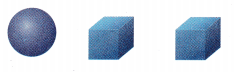Explanation:
Circled the similar
two cubes are similar

Question 2.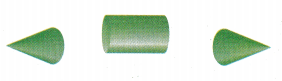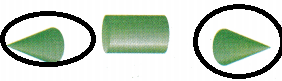Explanation:
Circled the similar
two cones are similar

Longer and Shorter

Circle the longer object.

Draw a line under the shorter object.

Question 3.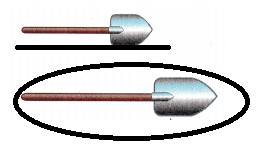Explanation:
Underlined the shorter one and
circled the longer

Question 4.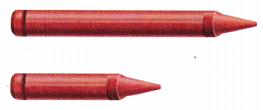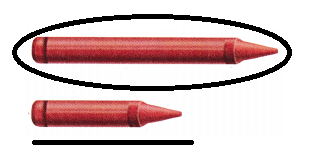Explanation:
Underlined the shorter one and
circled the longer

Identify Two-Dimensional Shapes

Color each square blue. Color each rectangle yellow. Color each circle red.

Question 5.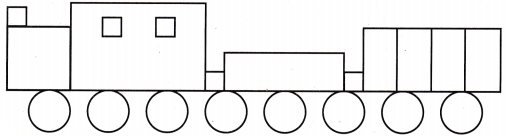Explanation:
Colored each square blue. Colored each rectangle yellow. Colored each circle red.

Family Note:

Vocabulary Builder

Visualize It

Write review words to name the solids.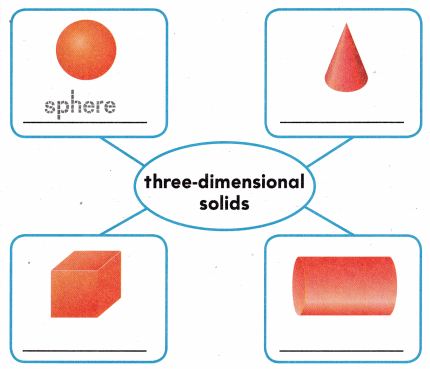Explanation: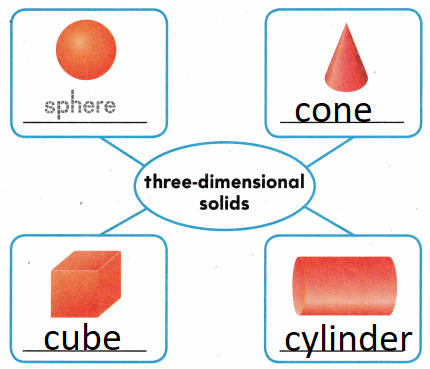Understand Vocabulary

Write the number of each shape.

Question 1.
__ circles
2 Circles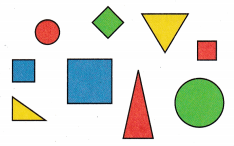Question 2.
___ squares
4 squares

Question 3.
___ triangles
3 triangles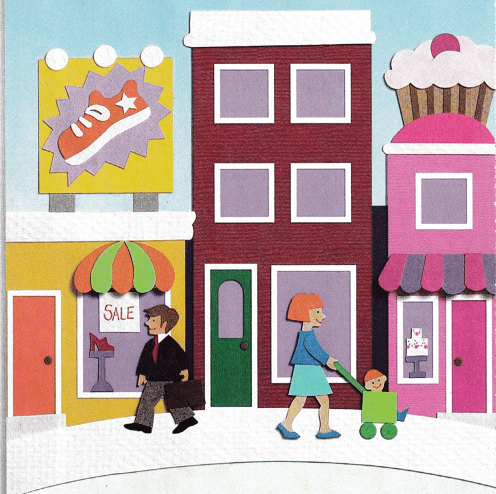We’re on our way to school.
We play a shape hunt. It’s so cool!
First find a square with equal sides, 1, 2, 3, 4.
Look! I spy one at the shoe store!

Find other figures shaped like a square.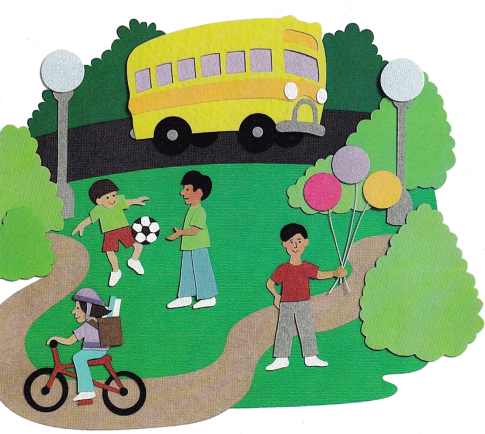The next figure that we look for
Is the circle. It’s so round.
Finding them is no chore,
For circles here abound.

Find figures shaped like a circle.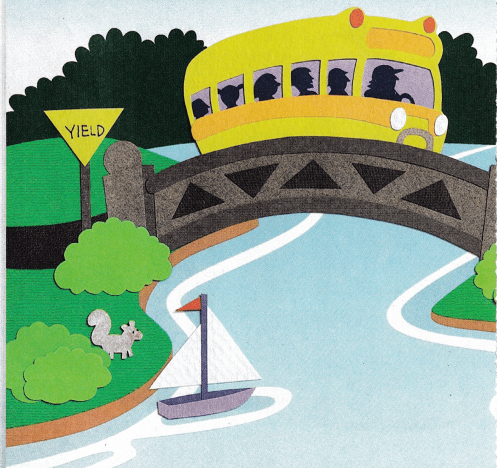Triangles are now next.
This figure has sides, 1,2, 3.
Now don’t be perplexed.
There are many here to see!

Find figures shaped like a triangle.We’re almost to our school,
As through the streets we wind.
Wait! We made a simple game rule
4 figures we must find!

Find figures shaped like a rectangle.We’re now here in our classroom,
At our desks, and in our chairs.
It was fun finding triangles,
circles, rectangles and squares.

Find and name these figures in our classroom.Explanation:Our game has ended, but this book is not done. Draw a city picture. Include all 4 figures just for fun!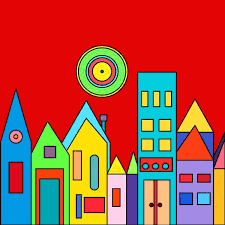Write Math Look at these shapes. Draw a picture, and write your own story about shapes in your classroom or outside.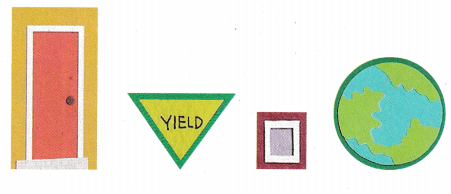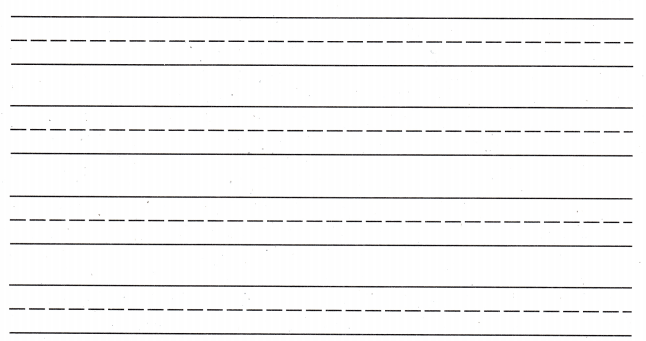Explanation:
The story in my own words by using the pictures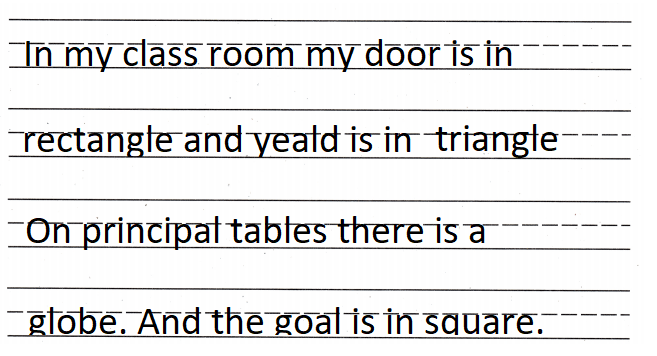The Shape of Things

Look at the shapes in the picture.

Question 1
How many squares can you find?
____ squares
5 squares
Explanation:
There are 5 squares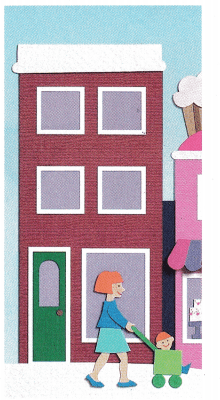Question 2.
How many circles can you find?
_______ circles
2 circle.
Explanation:
There are 2 circle.

Question 3.
How many rectangles can you find?
____ rectangles
5 rectangles
Explanation:
There are 5 rectangles

Question 4.
How many triangles can you find?
____ triangles